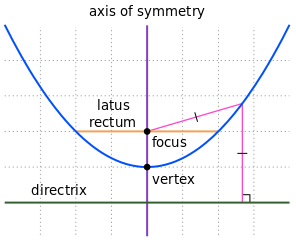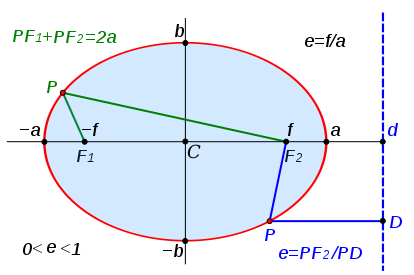Curves & Lines Math   Imaginary math   Trigonometry   Calculus Area & Volume ```Line Slope intercept y = mx + k m is slope, Δy/Δx Point-slope form y – y₁ = m(x – x₁) Two points form y – y₁ = (x – x₁)(y₂ – y₁) / (x₂ – x₁) Intercept form (x/a) + (y/b) = 1 a is x intercept b is y intercept Standard Form: the standard form of a line is in the form Ax + By = C where A is a positive integer, and B, and C are integers. Two lines are perpendicular if the product of their slopes is −1 or one has a slope of 0 (a horizontal line) and the other has an undefined slope (a vertical line). distance between two points d = √(Δx² + Δy² + Δz²) Distance point to line line is ax + by + c = 0 point is x₀, y₀ distance is |ax₀ + by₀ + c| / √(a² + b²) Angles Complementary angles add up to 90º or π/2 Supplimentary angles add up to 180º or π explementary angles add up to 360º or 2π acute angles are <90º right angle is 90º obtuse angles are >90º and <180º straight angle is 180º oblique angles are those not n•90º Circle equation of circle with center at a,b and radius r (x–a)² + (y–b)² = r² center at the origin x² + y² = r² three point form of a circle is: [ (x–x₁)(x–x₂) + (y–y₁)(y–y₂) ] / [ (y–y₁)(x–x₂) – (y–y₂)(x–x₁) ] = [(x₃–x₁)(x₃–x₂) + (y₃–y₁)(y₃–y₂)] / [(y₃–y₁)(x₃–x₂) – (y₃–y₂)(x₃–x₁)] A = πr² length of chord L = 2√(r²-d²) r = radius d = perpendicular distance from chord to center Area of a segment A = (r²/2)[(π/180)θ – sinθ] Plane General equation of a plane: ax + by + cz + d = 0 if d=0, plane goes thru the origin if x₀ y₀ z₀ are where plane intersects the 3 axes... x₀a = y₀b = z₀c = –d use this to solve for a,b,c,d sphere equation of sphere with center at a,b,c and radius r (x–a)² + (y–b)² + (z–c)² = r² center at the origin x² + y² + z² = r² The equation of the sphere centred at (x₀,y₀,z₀) and passing through (x₁,y₁,z₁) is (x-x₀)²+(y-y₀)²+(z-z₀)² = (x₁-x₀)²+(y₁-y₀)²+(z₁-z₀)² ``` ParabolaParabola vertical 4p(y–k) = (x–h)² p is distance vertex to focus, if positive opens up p is also distance vertex to directorix vertex at (h, k) Parabola open sideways 4p(x–h) = (y–k)² p is distance vertex to focus, if positive opens to the right p is also distance vertex to directorix vertex at (h, k) EllipseThe area is πab where a and b are one-half of the ellipse's major and minor axes and are the vertices. F₁ and F₂. The sum of the distances from any point P on the ellipse to those two foci is constant and equal to the major axis (PF₁ + PF₂ = 2a) foci are at a distance c from origin along the x axis, where c² = a² – b² Ellipse whose axis correspond to the x and y axis has the equation (x/a)² + (y/b)² = 1 a & b are distance from origin to curve along x or y axis. Ellipse centered at x₁ and y₁, the equation is ((x – x₁)/a)² + ((y – y₁)/b)² = 1 a & b are distance from center to curve along x or y axis. HyperbolaThe hyperbola consists of the red curves. The asymptotes of the hyperbola are shown as blue dashed lines and intersect at the center of the hyperbola, C. The two focal points are labeled F1 and F2, and the thin black line joining them is the transverse axis. The perpendicular thin black line through the center is the conjugate axis. The two thick black lines parallel to the conjugate axis (thus, perpendicular to the transverse axis) are the two directrices, D1 and D2. The eccentricity e equals the ratio of the distances from a point P on the hyperbola to one focus and its corresponding directrix line (shown in green). The two vertices are located on the transverse axis at ±a relative to the center. So the parameters are: a — distance from center C to either vertex b — length of a segment perpendicular to the transverse axis drawn from each vertex to the asymptotes c — distance from center C to either Focus point, F1 and F2, and θ — angle formed by each asymptote with the transverse axis. The shape of a hyperbola is defined entirely by its eccentricity ε, which is a dimensionless number always greater than one. The distance c from the center to the foci equals aε. The eccentricity can also be defined as the ratio of the distances to either focus and to a corresponding line known as the directrix; hence, the distance from the center to the directrices equals a/ε. In terms of the parameters a, b, c and the angle θ, the eccentricity ε equals ε = c/a = √(1 + (b²/a²)) = secθ For example, the eccentricity ε of a rectangular hyperbola (θ = 45°, a = b) equals √2. East-West opening hyperbola, ie, the transverse axis of any hyperbola is aligned with the x-axis and is centered on the origin (x²/a²) – (y²/b²) = 1 North–South opening hyperbola, ie, the transverse axis of any hyperbola is aligned with the y-axis and is centered on the origin (y²/a²) – (x²/b²) = 1 Home Area, Volume Atomic Mass Black Body Radiation Boolean Algebra Calculus Capacitor Center of Mass Carnot Cycle Charge Chemistry   Elements   Reactions Circuits Complex numbers Constants Curves, lines deciBell Density Electronics Elements Flow in fluids Fourier's Law Gases Gravitation Greek Alphabet Horizon Distance Interest Magnetics Math   Trig Math, complex Maxwell's Eq's Motion Newton's Laws Octal/Hex Codes Orbital Mechanics Particles Parts, Analog IC   Digital IC   Discrete Pendulum Planets Pressure Prime Numbers Questions Radiation Refraction Relativistic Motion Resistance, Resistivity Rotation Series SI (metric) prefixes Skin Effect Specific Heat Springs Stellar magnitude Thermal Thermal Conductivity Thermal Expansion Thermodynamics Trigonometry Units, Conversions Vectors Volume, Area Water Wave Motion Wire, Cu   Al   metric Young's Modulus